# NCERT Solutions for Class 5 Maths How Many Square## myCBSEguide App

CBSE, NCERT, JEE Main, NEET-UG, NDA, Exam Papers, Question Bank, NCERT Solutions, Exemplars, Revision Notes, Free Videos, MCQ Tests & more.

NCERT solutions for Class 5 Mathematics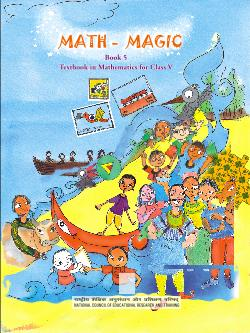## NCERT Solutions for Class 5 Maths How Many Square

###### PRACTICE TIME:

1. Ziri tried to make some other tiles. She started with a square of 2 cm side and made shapes like these. Look at these carefully and find out:

Q. Which of these shapes will tile a floor (without any gaps)? Discuss. What is the area of these shapes?

Q. Make designs in your copy by tiling those shapes.

Q. Now you create your own new tiles out of a square. Can you do the same with a triangle? Try doing it.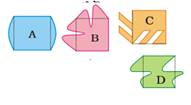Ans. The shapes C and D will tile a floor (without any gaps)

The area of each of these shapes (i.e. tiles) is

2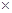2 square cm = 4 square cm.

NCERT Solutions for Class 5 Maths How Many Square

###### 2. Make a pattern using your tile. Trace the shape to repeat it on a page, but remember there must be no gaps between them. Ziri made a pattern using her yellow tiles. (You know the area of her tile.)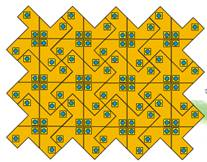Q. How many tiles has she used?

Q. What is the area of the floor pattern Ziri has made here?

Ans. She has used 12 tiles.

The area of the floor pattern Ziri has made here is (123) square cm= 36 square cm.

NCERT Solutions for Class 5 Maths How Many Square

###### 3. Did you get all the 12 shapes using 5 squares?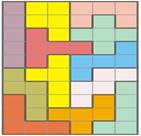Draw all the 12 shapes on a sheet of cardboard and cut them.

Ans. Yes, I get all the 12 shapes using 5 squares as shown above.

All the 12 shapes may be drawn on a sheet of cardboard and also cut them as desired.

NCERT Solutions for Class 5 Maths How Many Square

###### 4. Ziri went to a shop and was surprised to see the different designs of tiles on the floor. Aren’t these beautiful! Can you find the tile which is repeated to make each of these floor patterns? Circle a tile in each pattern.

Ans. A tile in each pattern is shown as encircle.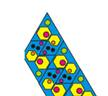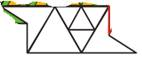NCERT Solutions for Class 5 Maths How Many Square

###### 5. After looking at the patterns Ziri wanted to make her own yellow tile. You too make a tile this way.

Step 1: Take a piece of cardboard or thick paper. Draw a square of side 3 cm on it.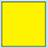Step 2: Draw a triangle on any one of the sides of this square.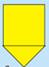Step 3: Draw another triangle of the same size on another side of the square. But this time draw it inside the square.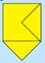Step 4: Cut this shape from the cardboard. Your tile is ready! What is it’s area?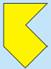Ans. It’s area is 33 square cm i.e. 9 square cm.

NCERT Solutions for Class 5 Maths How Many Square

###### 6. Here is a rectangle of area 20 square cm.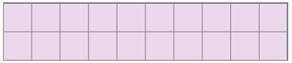a) Draw one straight line in this rectangle to divide it into two equal triangles. What is the area of each of the triangles?

b) Draw one straight line in this rectangle to divide it into two equal rectangles. What is the area of each of the smaller rectangles?

c) Draw two straight lines in this rectangle to divide it into one rectangle and two equal triangles.

d) What is the area of the rectangle?

e)  What is the area of each of the triangles?

Ans. (a) One straight line is drawn in the given rectangle to divide it in two equal triangle as: shown below.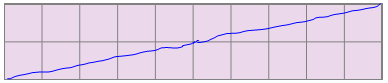Area of each of the triangles is half of the given rectangle. Area of this rectangle is 20 square cm. So, the area of each of the triangles is 10 squares cm.

(b) One straight line is drawn in the given rectangle to divide it into two equal rectangles as shown below: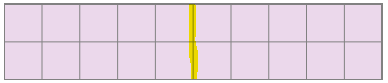Area of each of the smaller rectangles is half of the given rectangle. Area of the given rectangle is 20 square cm. So, the area of each of two rectangles is 10 square cm.

(c) Two straight lines are drawn in the given rectangle to divide it into one rectangle and two equal triangles as shown below: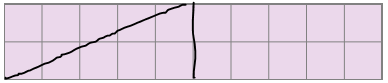(d) Area of the new rectangle is half of the given rectangle. Area of the given rectangle is 20 square cm. So, the area of the smaller rectangle is 10 square cm.

(e) Area of the each of the triangles is half of the rectangle of area 10 square cm. So, the area of each of the triangles is 5 square cm.

## NCERT Solutions for Class 5 Mathematics Chapter 5 Be My Multiple

NCERT Solutions Class 5 Maths PDF (Download) Free from myCBSEguide app and myCBSEguide website. Ncert solution class 5 Maths includes text book solutions from Class 5 Maths Book . NCERT Solutions for CBSE Class 5 Maths have total 14 chapters. 5 Maths NCERT Solutions in PDF for free Download on our website. Ncert Maths class 5 solutions PDF and Maths ncert class 5 PDF solutions with latest modifications and as per the latest CBSE syllabus are only available in myCBSEguide.

## CBSE app for Students

To download NCERT Solutions for Class 5 Maths, Social Science Computer Science, Home Science, Hindi English, Maths Science do check myCBSEguide app or website. myCBSEguide provides sample papers with solution, test papers for chapter-wise practice, NCERT solutions, NCERT Exemplar solutions, quick revision notes for ready reference, CBSE guess papers and CBSE important question papers. Sample Paper all are made available through the best app for CBSE students and myCBSEguide website.